# Equivalent Fractions, Part 1

Page 1 of 2
Check out these circles: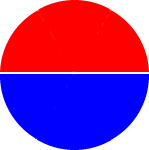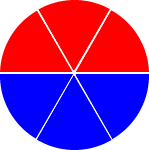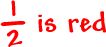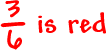The first one says that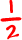is red and the second one says that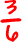is red... But, the same amount is red on both circles!

So,must be the same amount as.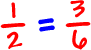These are called equivalent fractions. (Since they are equal amounts.)

Look at another one: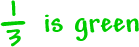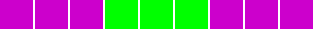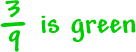The same amount is green on both so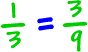You'll see more of this in the Equivalent Fractions Part 2 lesson, but here's a preview:

If you multiply the numerator and denominator of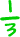by 3, you get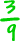.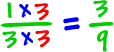Cool, huh?

Head on over to the next page to try some fraction practice problems...